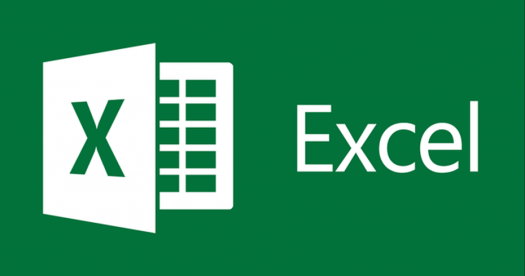# CMP 540 Excel Trivia Questions Quiz

8 QuestionsSettingsAfter taking the Excel tutorial, take this quiz.

Related Topics
• 1.
• A.

Calculations can be done automatically.

• B.

Changing data automatically updates calculations (as long as Excel is not set to calculate manually).

• C.

More flexibility.

• D.

All of the above.

• 2.
The intersection of a row and a column is called:
• A.

Data.

• B.

A field.

• C.

A cell.

• D.

An equation.

• 3.
• A.

The first cell referenced.

• B.

Parenthesis.

• C.

Quotation marks.

• D.

An equal sign.

• 4.
The cell labeled F5 refers to:
• A.

Row F column 5.

• B.

Column F row 5.

• C.

Functions available in cells.

• D.

Function key F4.

• 5.
There are three types of data found in a spreadsheet:
• A.

Data, words, numbers

• B.

Equations, data, numbers

• C.

Words, numbers, labels

• D.

Numbers, formulas, labels

• 6.
The formula =B2+A3 is located in cell b3. If this was copied and pasted into cell D4 the resulting formula would be:
• A.

=C2+C3

• B.

=D3+C3

• C.

=D3+C4

• D.

None of the above

• 7.
• A.

=\$c\$3*e3

• B.

=c3*e3

• C.

=\$c\$3*d3

• D.

24

• 8.
A constant is another name for this type of data:
• A.

Number

• B.

Equation

• C.

Formula

• D.

Description